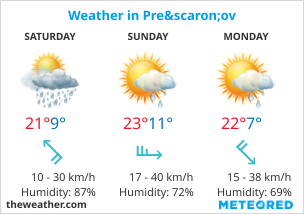SlovakiaCelsius FahrenheitYou are here.  Home  >  Blog  >  How many onions should I cut

# How many onions should I cut

## 1 large onion equals 8 small ones

September 1, 2019,  |  Often we can't imagine how many small onions we should cut instead of the big one. Luckily, we can calculate this using a simple three-dimensional sphere volume formula. Suppose we have a large onion that has each of 3 dimensions twice the size of the small one. If the radius r is, for example, 2 cm, then the radius of the large onion 2r is 4 cm. The volume of a large sphere is always 8 times greater than the volume of a sphere with a half radius r.
V1 = 4 / 3 ˣ π ˣ (2r)3
V2 = 4 / 3 ˣ π ˣ r3
V1 = 4 / 3 ˣ 3,14 ˣ (4 cm)3
V2 = 4 / 3 ˣ 3,14 ˣ (2 cm)3
V1 = 4,19 ˣ 64 cm3
V2 = 4,19 ˣ 8 cm3V1: Volume of sphere / large onion
V2: Volume of sphere / small onion
r1: 2r - Radius of a large onion
r2: r - Radius of a small onion
π: 3,14 - Ludolf's number

V1 = 4 / 3 ˣ 3,14 ˣ 2r ˣ 2r ˣ 2r
V2 = 4 / 3 ˣ 3,14 ˣ r ˣ r ˣ r

V1 = 4,19 ˣ 8r3
V2 = 4,19 ˣ r3

V1 = 8 ˣ V2

 Simplified view Dark mode
By accessing vla.sk pages, you agree to abide by Terms and conditions of use.